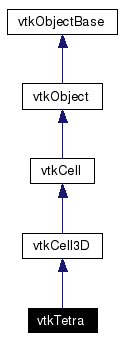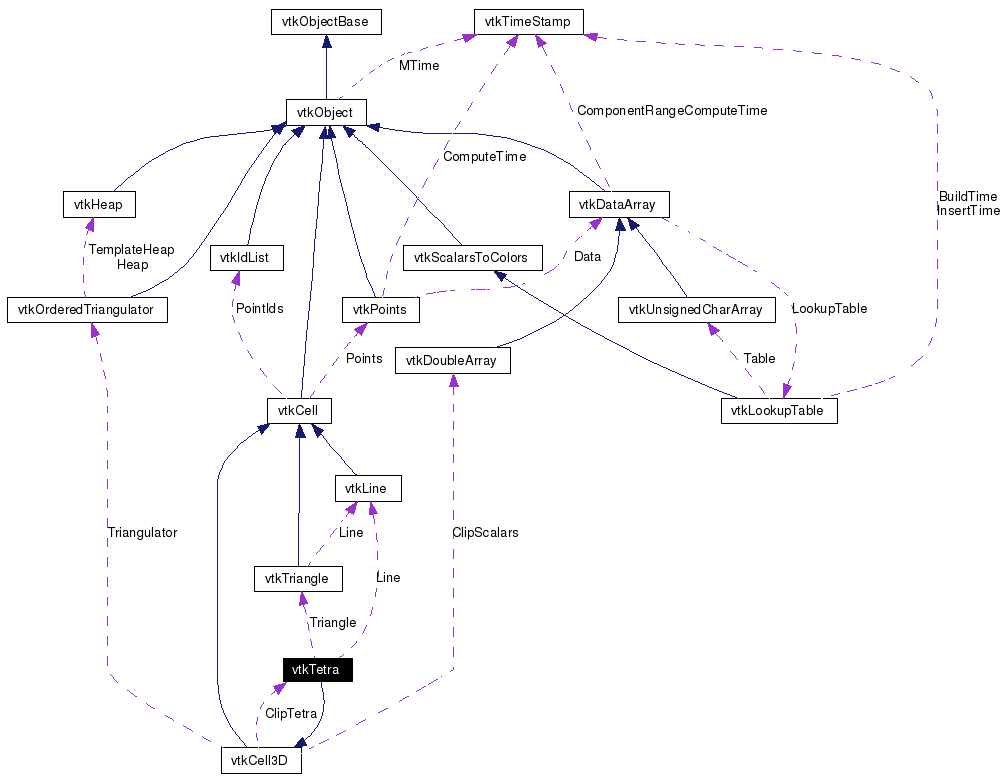# vtkTetra Class Reference

`#include <vtkTetra.h>`

Inheritance diagram for vtkTetra:[legend]
Collaboration diagram for vtkTetra:[legend]
List of all members.

## Detailed Description

a 3D cell that represents a tetrahedron

vtkTetra is a concrete implementation of vtkCell to represent a 3D tetrahedron. vtkTetra uses the standard isoparametric shape functions for a linear tetrahedron. The tetrahedron is defined by the four points (0-3); where (0,1,2) is the base of the tetrahedron which, using the right hand rule, forms a triangle whose normal points in the direction of the fourth point.

vtkConvexPointSet vtkHexahedron vtkPyramid vtkVoxel vtkWedge
Examples:
vtkTetra (Examples)
Tests:
vtkTetra (Tests)

Definition at line 44 of file vtkTetra.h.
double GetParametricDistance (double pcoords)
static void TetraCenter (double p1, double p2, double p3, double p4, double center)
int JacobianInverse (double **inverse, double derivs)
static void InterpolationFunctions (double pcoords, double weights)
static void InterpolationDerivs (double derivs)
static int * GetEdgeArray (int edgeId)
static int * GetFaceArray (int faceId)

## Public Types

typedef vtkCell3D Superclass

## Public Member Functions

virtual const char * GetClassName ()
virtual int IsA (const char *type)
int CellBoundary (int subId, double pcoords, vtkIdList *pts)
int GetParametricCenter (double pcoords)
void PrintSelf (ostream &os, vtkIndent indent)
virtual void GetEdgePoints (int edgeId, int *&pts)
virtual void GetFacePoints (int faceId, int *&pts)
int GetCellType ()
int GetNumberOfEdges ()
int GetNumberOfFaces ()
vtkCellGetEdge (int edgeId)
vtkCellGetFace (int faceId)
void Contour (double value, vtkDataArray *cellScalars, vtkPointLocator *locator, vtkCellArray *verts, vtkCellArray *lines, vtkCellArray *polys, vtkPointData *inPd, vtkPointData *outPd, vtkCellData *inCd, vtkIdType cellId, vtkCellData *outCd)
void Clip (double value, vtkDataArray *cellScalars, vtkPointLocator *locator, vtkCellArray *connectivity, vtkPointData *inPd, vtkPointData *outPd, vtkCellData *inCd, vtkIdType cellId, vtkCellData *outCd, int insideOut)
int EvaluatePosition (double x, double *closestPoint, int &subId, double pcoords, double &dist2, double *weights)
void EvaluateLocation (int &subId, double pcoords, double x, double *weights)
int IntersectWithLine (double p1, double p2, double tol, double &t, double x, double pcoords, int &subId)
int Triangulate (int index, vtkIdList *ptIds, vtkPoints *pts)
void Derivatives (int subId, double pcoords, double *values, int dim, double *derivs)
virtual double * GetParametricCoords ()

## Static Public Member Functions

static vtkTetraNew ()
static int IsTypeOf (const char *type)
static vtkTetraSafeDownCast (vtkObject *o)
static double Circumsphere (double p1, double p2, double p3, double p4, double center)
static double Insphere (double p1, double p2, double p3, double p4, double center)
static int BarycentricCoords (double x, double x1, double x2, double x3, double x4, double bcoords)
static double ComputeVolume (double p1, double p2, double p3, double p4)

vtkTetra ()
~vtkTetra ()

## Protected Attributes

vtkLineLine
vtkTriangleTriangle

## Member Typedef Documentation

 typedef vtkCell3D vtkTetra::Superclass
 Reimplemented from vtkCell3D. Definition at line 48 of file vtkTetra.h.

## Constructor & Destructor Documentation

 vtkTetra::vtkTetra ( ) ` [protected]`

 vtkTetra::~vtkTetra ( ) ` [protected]`

## Member Function Documentation

 static vtkTetra* vtkTetra::New ( ) ` [static]`
 Create an object with Debug turned off, modified time initialized to zero, and reference counting on. Reimplemented from vtkObject.

 virtual const char* vtkTetra::GetClassName ( ) ` [virtual]`
 Reimplemented from vtkCell3D.

 static int vtkTetra::IsTypeOf ( const char * type ) ` [static]`
 Return 1 if this class type is the same type of (or a subclass of) the named class. Returns 0 otherwise. This method works in combination with vtkTypeRevisionMacro found in vtkSetGet.h. Reimplemented from vtkCell3D.

 virtual int vtkTetra::IsA ( const char * type ) ` [virtual]`
 Return 1 if this class is the same type of (or a subclass of) the named class. Returns 0 otherwise. This method works in combination with vtkTypeRevisionMacro found in vtkSetGet.h. Reimplemented from vtkCell3D.

 static vtkTetra* vtkTetra::SafeDownCast ( vtkObject * o ) ` [static]`
 Reimplemented from vtkCell3D.

 void vtkTetra::PrintSelf ( ostream & os, vtkIndent indent ) ` [virtual]`
 Methods invoked by print to print information about the object including superclasses. Typically not called by the user (use Print() instead) but used in the hierarchical print process to combine the output of several classes. Reimplemented from vtkCell3D.

 virtual void vtkTetra::GetEdgePoints ( int edgeId, int *& pts ) ` [virtual]`
 See vtkCell3D API for description of these methods. Implements vtkCell3D.

 virtual void vtkTetra::GetFacePoints ( int faceId, int *& pts ) ` [virtual]`
 Get the list of vertices that define a face. The list is terminated with a negative number. Note that the vertices are 0-offset; that is, they refer to the ids of the cell, not the point ids of the mesh that the cell belongs to. The faceId must range between 0<=faceIdGetNumberOfFaces(). Implements vtkCell3D.

 int vtkTetra::GetCellType ( ) ` [inline, virtual]`
 See the vtkCell API for descriptions of these methods. Implements vtkCell. Definition at line 59 of file vtkTetra.h. References VTK_TETRA.

 int vtkTetra::GetNumberOfEdges ( ) ` [inline, virtual]`
 See the vtkCell API for descriptions of these methods. Implements vtkCell. Definition at line 60 of file vtkTetra.h.

 int vtkTetra::GetNumberOfFaces ( ) ` [inline, virtual]`
 See the vtkCell API for descriptions of these methods. Implements vtkCell. Definition at line 61 of file vtkTetra.h.

 vtkCell* vtkTetra::GetEdge ( int edgeId ) ` [virtual]`
 See the vtkCell API for descriptions of these methods. Implements vtkCell.

 vtkCell* vtkTetra::GetFace ( int faceId ) ` [virtual]`
 See the vtkCell API for descriptions of these methods. Implements vtkCell.

 void vtkTetra::Contour ( double value, vtkDataArray * cellScalars, vtkPointLocator * locator, vtkCellArray * verts, vtkCellArray * lines, vtkCellArray * polys, vtkPointData * inPd, vtkPointData * outPd, vtkCellData * inCd, vtkIdType cellId, vtkCellData * outCd ) ` [virtual]`
 See the vtkCell API for descriptions of these methods. Reimplemented from vtkCell3D.

 void vtkTetra::Clip ( double value, vtkDataArray * cellScalars, vtkPointLocator * locator, vtkCellArray * connectivity, vtkPointData * inPd, vtkPointData * outPd, vtkCellData * inCd, vtkIdType cellId, vtkCellData * outCd, int insideOut ) ` [virtual]`
 See the vtkCell API for descriptions of these methods. Reimplemented from vtkCell3D.

 int vtkTetra::EvaluatePosition ( double x, double * closestPoint, int & subId, double pcoords, double & dist2, double * weights ) ` [virtual]`
 See the vtkCell API for descriptions of these methods. Implements vtkCell.

 void vtkTetra::EvaluateLocation ( int & subId, double pcoords, double x, double * weights ) ` [virtual]`
 See the vtkCell API for descriptions of these methods. Implements vtkCell.

 int vtkTetra::IntersectWithLine ( double p1, double p2, double tol, double & t, double x, double pcoords, int & subId ) ` [virtual]`
 See the vtkCell API for descriptions of these methods. Implements vtkCell.

 int vtkTetra::Triangulate ( int index, vtkIdList * ptIds, vtkPoints * pts ) ` [virtual]`
 See the vtkCell API for descriptions of these methods. Implements vtkCell.

 void vtkTetra::Derivatives ( int subId, double pcoords, double * values, int dim, double * derivs ) ` [virtual]`
 See the vtkCell API for descriptions of these methods. Implements vtkCell.

 virtual double* vtkTetra::GetParametricCoords ( ) ` [virtual]`
 See the vtkCell API for descriptions of these methods. Reimplemented from vtkCell.

 int vtkTetra::CellBoundary ( int subId, double pcoords, vtkIdList * pts ) ` [virtual]`
 Returns the set of points that are on the boundary of the tetrahedron that are closest parametrically to the point specified. This may include faces, edges, or vertices. Implements vtkCell.

 int vtkTetra::GetParametricCenter ( double pcoords ) ` [inline, virtual]`
 Return the center of the tetrahedron in parametric coordinates. Reimplemented from vtkCell. Definition at line 169 of file vtkTetra.h.

 double vtkTetra::GetParametricDistance ( double pcoords ) ` [virtual]`
 Return the distance of the parametric coordinate provided to the cell. If inside the cell, a distance of zero is returned. Reimplemented from vtkCell.

 static void vtkTetra::TetraCenter ( double p1, double p2, double p3, double p4, double center ) ` [static]`
 Compute the center of the tetrahedron,

 static double vtkTetra::Circumsphere ( double p1, double p2, double p3, double p4, double center ) ` [static]`
 Compute the circumcenter (center) and radius squared (method return value) of a tetrahedron defined by the four points x1, x2, x3, and x4.

 static double vtkTetra::Insphere ( double p1, double p2, double p3, double p4, double center ) ` [static]`
 Compute the center (center) and radius (method return value) of a sphere that just fits inside the faces of a tetrahedron defined by the four points x1, x2, x3, and x4.

 static int vtkTetra::BarycentricCoords ( double x, double x1, double x2, double x3, double x4, double bcoords ) ` [static]`
 Given a 3D point x, determine the barycentric coordinates of the point. Barycentric coordinates are a natural coordinate system for simplices that express a position as a linear combination of the vertices. For a tetrahedron, there are four barycentric coordinates (because there are four vertices), and the sum of the coordinates must equal 1. If a point x is inside a simplex, then all four coordinates will be strictly positive. If three coordinates are zero (so the fourth =1), then the point x is on a vertex. If two coordinates are zero, the point x is on an edge (and so on). In this method, you must specify the vertex coordinates x1->x4. Returns 0 if tetrahedron is degenerate.

 static double vtkTetra::ComputeVolume ( double p1, double p2, double p3, double p4 ) ` [static]`
 Compute the volume of a tetrahedron defined by the four points p1, p2, p3, and p4.

 int vtkTetra::JacobianInverse ( double ** inverse, double derivs )
 Given parametric coordinates compute inverse Jacobian transformation matrix. Returns 9 elements of 3x3 inverse Jacobian plus interpolation function derivatives. Returns 0 if no inverse exists.

 static void vtkTetra::InterpolationFunctions ( double pcoords, double weights ) ` [static]`
 Tetra specific methods.

 static void vtkTetra::InterpolationDerivs ( double derivs ) ` [static]`
 Given parametric coordinates compute inverse Jacobian transformation matrix. Returns 9 elements of 3x3 inverse Jacobian plus interpolation function derivatives. Returns 0 if no inverse exists.

 static int* vtkTetra::GetEdgeArray ( int edgeId ) ` [static]`
 Given parametric coordinates compute inverse Jacobian transformation matrix. Returns 9 elements of 3x3 inverse Jacobian plus interpolation function derivatives. Returns 0 if no inverse exists.

 static int* vtkTetra::GetFaceArray ( int faceId ) ` [static]`
 Given parametric coordinates compute inverse Jacobian transformation matrix. Returns 9 elements of 3x3 inverse Jacobian plus interpolation function derivatives. Returns 0 if no inverse exists.

## Member Data Documentation

 vtkLine* vtkTetra::Line` [protected]`
 Definition at line 161 of file vtkTetra.h.

 vtkTriangle* vtkTetra::Triangle` [protected]`
 Definition at line 162 of file vtkTetra.h.

The documentation for this class was generated from the following file:

Generated on Tue Jan 22 00:21:34 2008 for VTK by1.4.3-20050530